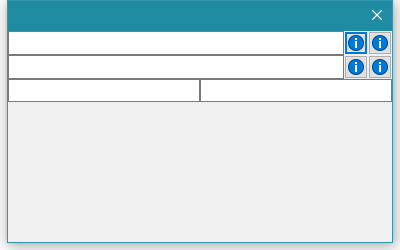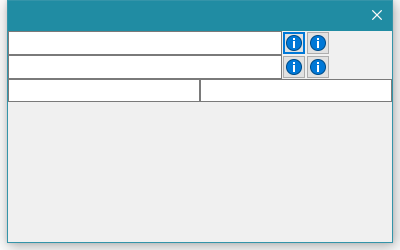# GridBagSizer: Wrong cell size with colspan?

Hello, I have a problem with the wx.GridBagSizer when aligning items.

This here is my dialog:

``````import wx

class TestFrameGridBag(wx.Dialog):
def __init__(self, parent):
super().__init__(parent)
szr = wx.GridBagSizer()
self.SetMinSize((50, 100))

self.btn1 = wx.BitmapButton(self, bitmap=wx.ArtProvider.GetBitmap(wx.ART_INFORMATION, size=(16, 16)))
self.btn2 = wx.BitmapButton(self, bitmap=wx.ArtProvider.GetBitmap(wx.ART_INFORMATION, size=(16, 16)))
self.btn3 = wx.BitmapButton(self, bitmap=wx.ArtProvider.GetBitmap(wx.ART_INFORMATION, size=(16, 16)))
self.btn4 = wx.BitmapButton(self, bitmap=wx.ArtProvider.GetBitmap(wx.ART_INFORMATION, size=(16, 16)))

szr.Add(wx.TextCtrl(self), (0, 0), flag=wx.EXPAND)
szr.Add(self.btn1, (0, 1))
szr.Add(self.btn2, (0, 2))

szr.Add(wx.TextCtrl(self), (1, 0), flag=wx.EXPAND)
szr.Add(self.btn3, (1, 1))
szr.Add(self.btn4, (1, 2))

pnl = wx.Panel(self)  # with size=(0, -1) it works like expected
bszr = wx.BoxSizer(wx.HORIZONTAL)
bszr.Add(wx.TextCtrl(pnl), proportion=1, flag=wx.EXPAND)
bszr.Add(wx.TextCtrl(pnl), proportion=1, flag=wx.EXPAND)
pnl.SetSizer(bszr)

szr.Add(pnl, (2, 0), span=(1, 3), flag=wx.EXPAND)  # with span=(1, 1) it works like expected, but it is too short.
szr.AddGrowableCol(0, 1)

self.SetSizer(szr)

app = wx.App()
dlg = TestFrameGridBag(None)
dlg.ShowModal()
``````

``

And this is what I want to get:However, this it what I do get:To me, it looks like the min size of the right column is calculated wrong.

As a workaround I can do the following things:

• Wrap the items at the bottom in a panel (as in the code example above), and set the size of this panel to (0, -1). However, if I do this.GetSizer().ComputeFittingWindowSize() returns wrong values, since the minimum width of the item at the bottom of the dialog is 0. Min sizes of elements that are wrapped by this panel are ignored. That makes it difficult to me to dynamically calculate the min size of that dialog.
• Alternatively, I can add more columns: If the elements in the first column have a colspan of 20 (so the buttons are in column 21 and 22), then I have a workaround for this problem too. But this seems very ugly to me too.
Now to my question: Is this a bug or is there any “nice” way to
1. Align the things like in the the first screenshot

2. Be able to calculate a “good” size for elements.

Thank you in advance and best regards